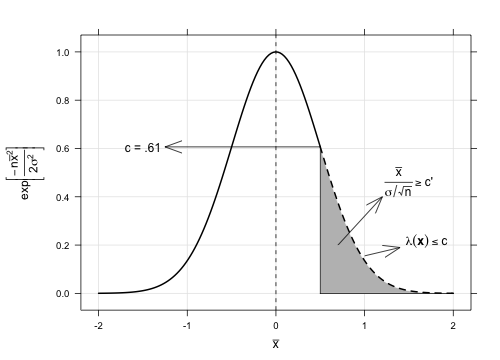# Blog Archives

## R and Python: Gradient Descent

December 22, 2015
By

One of the problems often dealt in Statistics is minimization of the objective function. And contrary to the linear models, there is no analytical solution for models that are nonlinear on the parameters such as logistic regression, neural networks, and nonlinear regression models (like Michaelis-Menten model). In this situation, we have to use mathematical programming or optimization. And one...

## R and Python: Theory of Linear Least Squares

December 15, 2015
ByIn my previous article, we talked about implementations of linear regression models in R, Python and SAS. On the theoretical sides, however, I briefly mentioned the estimation procedure for the parameter \$boldsymbol{beta}\$. So to help us understand how software does the estimation procedure, we'll look at the mathematics behind it. We will also perform the estimation manually in R...

## R, Python, and SAS: Getting Started with Linear Regression

August 16, 2015
ByConsider the linear regression model, \$\$ y_i=f_i(boldsymbol{x}|boldsymbol{beta})+varepsilon_i, \$\$ where \$y_i\$ is the response or the dependent variable at the \$i\$th case, \$i=1,cdots, N\$ and the predictor or the independent variable is the \$boldsymbol{x}\$ term defined in the mean function \$f_i(boldsymbol{x}|boldsymbol{beta})\$. For simplicity, consider the following simple linear regression (SLR) model, \$\$ y_i=beta_0+beta_1x_i+varepsilon_i. \$\$ To obtain the (best) estimate of...

## Parametric Inference: Karlin-Rubin Theorem

July 20, 2015
ByA family of pdfs or pmfs \${g(t|theta):thetainTheta}\$ for a univariate random variable \$T\$ with real-valued parameter \$theta\$ has a monotone likelihood ratio (MLR) if, for every \$theta_2__theta_1\$, \$g(t|theta_2)/g(t|theta_1)\$ is a monotone (nonincreasing or nondecreasing) function of \$t\$ on \${t:g(t|theta_1)__0;text{or};g(t|theta_2)__0}\$. Note that \$c/0\$ is defined as \$infty\$ if \$0__ c\$. Consider testing \$H_0:thetaleq theta_0\$ versus \$H_1:theta__theta_0\$. Suppose that \$T\$ is...

## Parametric Inference: Likelihood Ratio Test Problem 2

May 23, 2015
ByMore on Likelihood Ratio Test, the following problem is originally from Casella and Berger (2001), exercise 8.12.ProblemFor samples of size \$n=1,4,16,64,100\$ from a normal population with mean \$mu\$ and known variance \$sigma^2\$, plot the power function of the following LRTs (Likelihood Ratio Tests). Take \$alpha = .05\$. \$H_0:muleq 0\$ versus \$H_1:mu__0\$\$H_0:mu=0\$ versus \$H_1:muneq 0\$SolutionThe LRT statistic is given by...

## Python and R: Basic Sampling Problem

April 16, 2015
By

In this post, I would like to share a simple problem about sampling analysis. And I will demonstrate how to solve this using Python and R. The first two problems are originally from Sampling: Design and Analysis book by Sharon Lohr.ProblemsLet \$N=6\$ and \$n=3\$. For purposes of studying sampling distributions, assume that all population values are known. \$y_1 =...

## R: How to Layout and Design an Infographic

February 26, 2015
ByAs promised from my recent article, here's my tutorial on how to layout and design an infographic in R. This article will serve as a template for more infographic design that I plan to share on future posts. Hence, we will go through the following sect...

## Philippine Infographic: Recapitulation on Incidents Involving Motorcycle Riding in Tandem Criminals for 2011-2013

February 18, 2015
ByThe Philippine government has launched Open Data Philippines (data.gov.ph) last year, January 16, 2014. Accordingly, the data.gov.ph aims to make national government data searchable, accessible, and useful, with the help of the different agencies of go...

## Canonical Correlation Analysis on Imaging

January 5, 2015
ByIn imaging, we deal with multivariate data, like in array form with several spectral bands. And trying to come up with interpretation across correlations of its dimensions is very challenging, if not impossible. For example let's recall the number of s...

## Principal Component Analysis on Imaging

December 25, 2014
ByEver wonder what's the mathematics behind face recognition on most gadgets like digital camera and smartphones? Well for most part it has something to do with statistics. One statistical tool that is capable of doing such feature is the Principal Component Analysis (PCA). In this post, however, we will not do (sorry to disappoint you) face recognition as we...